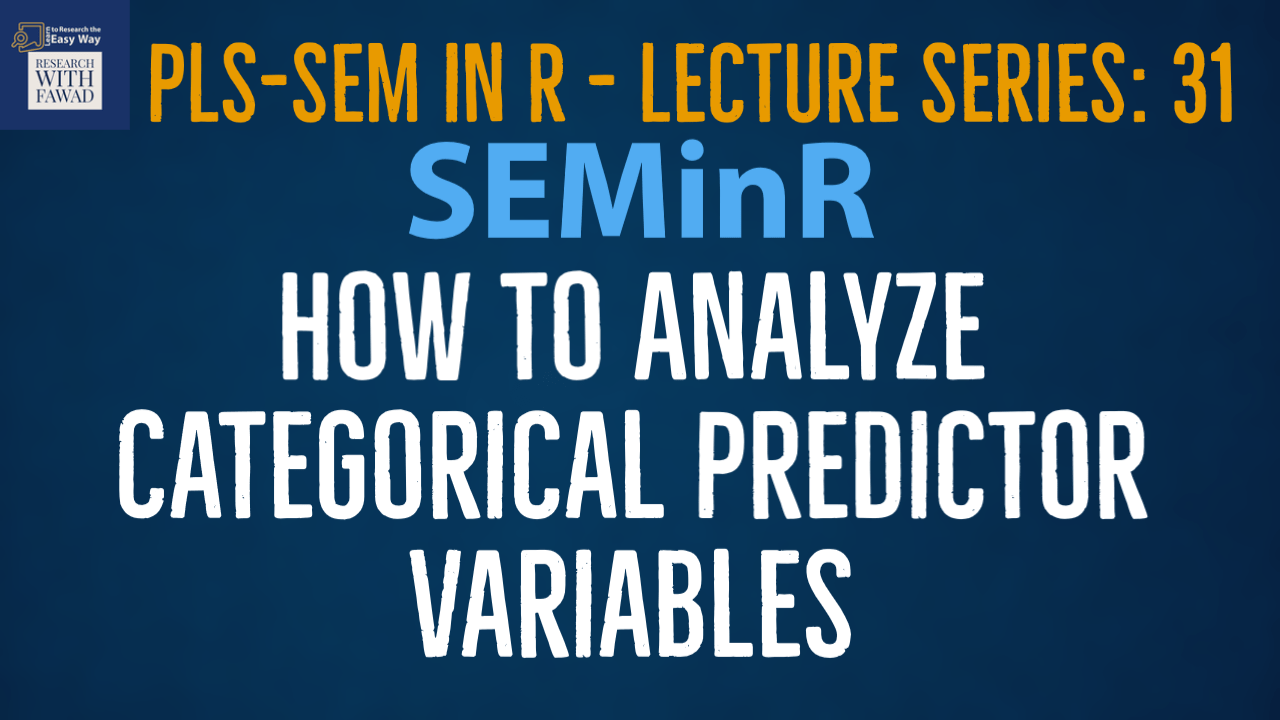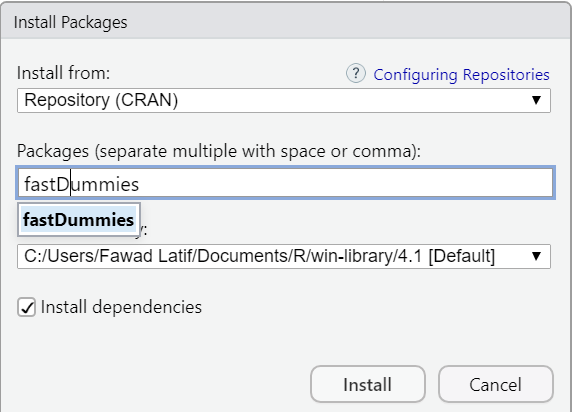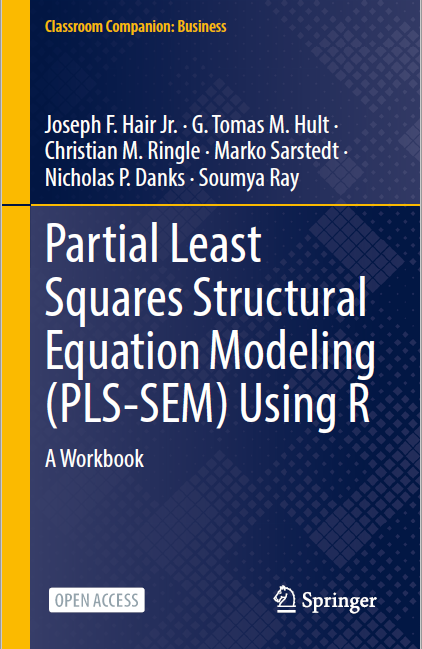# SEMinR Lecture Series - Analyzing Categorical Predictors### SEMinR Lecture Series

This session is focused on how to perform moderation analysis using SEMinR in Cran R.

## Categorical Predictor Variables using SEMinR in R

• The objective is to assess whether Country has an impact on Customer Loyalty.
• Country is a categorical variable in the study with three countries China, Pakistan, and Italy.
• Country is not added into the model directly. Since, it is a categorical variable, first the variable is dummy coded. Each Country will become a separate variable.
• In this case Two categories (Countries) will be added in the model estimation. Whereas the third country will serve as a reference category.## Create Dummy Variables in R

• Dummy variables are created when the exogenous variable is categorical in nature.
• Each category is transformed into a dummy variable.
• To create dummy variables in R, install fastDummies package## Complete Code

``````library(seminr)
library(fastDummies)
# Create dummy variables
datas <- dummy_cols(datas, select_columns = "Country")
# Create measurement model
simple_mm <- constructs(
composite("Loyalty", multi_items("CL", 1:6)),
composite("Pakistan", single_item("Country_2")),
composite("Italy", single_item("Country_3")))
# Create structural model
simple_sm <- relationships(
paths(from = c("Pakistan", "Italy"), to = "Loyalty"))
# Estimate the model
simple_model <- estimate_pls(data = datas,
measurement_model = simple_mm,
structural_model = simple_sm,
missing = mean_replacement,
missing_value = "-99")
# Summarize the model results
summary_simple <- summary(simple_model)
summary_simple
# Bootstrap the model on the PLS Estimated Model
boot_model <- bootstrap_model(
seminr_model = simple_model,
nboot = 5000,
cores = parallel::detectCores(),
seed = 123)
# Store the summary of the bootstrapped model
# alpha sets the specified level for significance, i.e. 0.05
# Inspect the bootstrapped structural paths
summary_boot\$bootstrapped_paths``````

## Reference

Hair Jr, J. F., Hult, G. T. M., Ringle, C. M., Sarstedt, M., Danks, N. P., & Ray, S. (2021). Partial Least Squares Structural Equation Modeling (PLS-SEM) Using R: A Workbook.The tutorials on SEMinR are based on the mentioned book. The book is open source and available for download under this link.